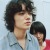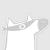AAAI 2020 将于美国纽约举办，时间在 2 月 7 日-2 月 12 日，本届大会将是第 34 届 AAAI 大会。 AAAI 的英文全称是 Association for the Advance of Artificial Intelligence——美国人工智能协会。该协会是人工智能领域的主要学术组织之一，其主办的年会也是人工智能领域的国际顶级会议。在中国计算机学会的国际学术会议排名以及清华大学新发布的计算机科学推荐学术会议和期刊列表中，AAAI 均被列为人工智能领域的 A 类顶级会议。0+0+0+0+0+0+0+0+0+0+0+0+0+0+0+0+0+0+0+4+0+0+0+0+0+0+0+0+0+0+0+0+0+0+0+0+0+0+0+0+0+0+0+0+0+
Top